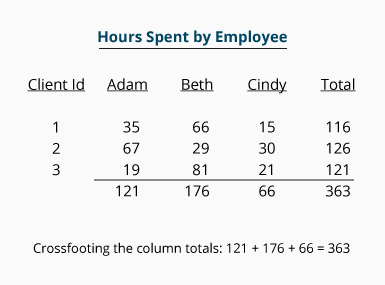# What does crossfoot mean?

## Definition of Crossfoot or Crossfooting

Accountants and auditors use the word foot to mean adding one or more columns of numbers. When there are several columns of numbers along with a "Total" column, they will crossfoot the totals. Crossfooting means to sum the total amounts appearing at the bottom of each column and verify that this "grand total" is equal to the total shown at the bottom of the "Total" column.

## Example of Crossfoot or Crossfooting

We will use the table below to illustrate the meaning of crossfoot or crossfooting. Note that the table shows the hours that three employees spent working for three clients. The column for Adam has the sum of 121, Beth's has 176, and Cindy's has 66. An auditor will "foot" each of these columns as well as the "Total" column to verify that the detailed amounts appearing in each column add up to the each column's sum (121, 176, 66, and 363).

In addition to summing or footing each column's detail, the auditor will crossfoot the totals of Adam's, Beth's, and Cindy's columns to be certain that the sum of these three column totals is equal to the sum of the "Total" column. In the following table crossfooting means adding 121 + 176 + 66 to be certain that its total of 363 is equal to the total or sum of the "Total" column's 363.### Free Debits and Credits Cheat Sheet

546,108
Subscribers
You are already subscribed. This offer is not available to existing subscribers.
Error: You have unsubscribed from this list.